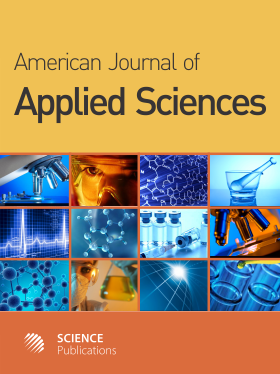Frequency: Continuous
ISSN: 1546-9239 (Print)
ISSN: 1554-3641 (Online)
Research Article Open Access

# Discovery of an Equilibrium Circle in the Circular Restricted Three Body Problem

Fawzy A. Abd El-Salam1
• 1 Department of Math, Faculty of Science, Taibah University, Al-Madeenah, Saudi Arabia

## Abstract

Problem statement: A traial to find equilibrium points in out of plane of the restricted three body problem. Approach: Solution of the equations of motion at equilibrium points. Linearizing the equations of motion and computing the eigen values to investigate the stability. Results: New triangular equilibrium points in plane perpendicular to the plane of motion and passing through Lagrange triangular equilibrium points are obtained. A circle of equilibrium points, namely Fawzy equilibrium circle is discovered. Infinite number of equilibrium points located on the circumference of this circle are computed. The obtained solutions are checked via obtaining some special cases. The stability of Fawzy equilibrium circle is studied. The oscillatory stable solutions as η-dependent and ξ-dependent stabilities are derived. Conclusion: The equilibrium points in out of plane of motion of the restricted three body problem is investigated. We obtained the following very new results:- First we obtained Fawzy ξξ-triangular equilibrium points in the plane η = 0. Second we obtained the so called Fawzy equilibrium circle. We checked our solutions via obtaining some special cases. We studied the stability of Fawzy equilibrium circle. We derived the oscillatory stable solutions as η-dependent and ξ-dependent stabilities. The stability of Lagrange as well as Fawzy triangular equilibrium points followed directly because they are subsets of the Fawzy equilibrium circle.

American Journal of Applied Sciences
Volume 9 No. 9, 2012, 1378-1384

DOI:

Submitted On: 16 January 2012 Published On: 16 July 2012

How to Cite: Abd El-Salam, F. A. (2012). Discovery of an Equilibrium Circle in the Circular Restricted Three Body Problem. American Journal of Applied Sciences, 9(9), 1378-1384. https://doi.org/10.3844/ajassp.2012.1378.1384

• 2,199 Views